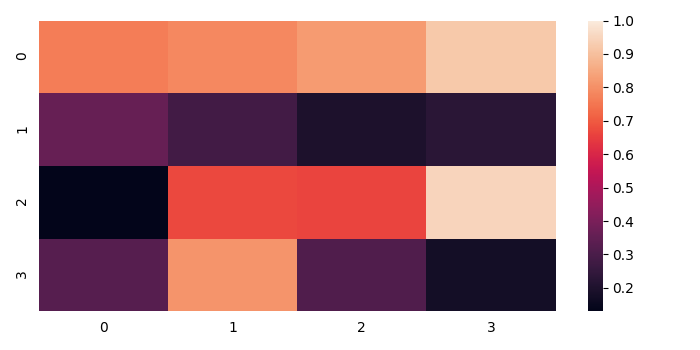# How to remove the axis tick marks on a Seaborn heatmap?

To remove the axis tick marks on a Seaborn heatmap, we can take the following steps

## Steps

• Set the figure size and adjust the padding between and around the subplots.

• Create random data points with 4×4 dimension.

• Plot the rectangular data as a color-encoded matrix.

• Use tick_params() for changing the appearance of ticks and tick labels. Use left=false and bottom=false to remove the tick marks.

• To display the figure, use Show() method.

## Example

import numpy as np
import seaborn as sns
from matplotlib import pyplot as plt

plt.rcParams["figure.figsize"] = [7.00, 3.50]
plt.rcParams["figure.autolayout"] = True

data = np.random.rand(4, 4)

ax = sns.heatmap(data, vmax=1)
ax.tick_params(left=False, bottom=False)

plt.show()

## Output

It will display the following Output −